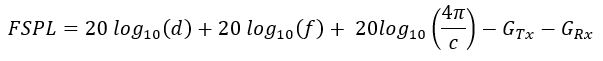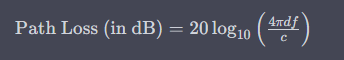# Path Loss CalculatorThe path loss formula is used to calculate the attenuation or loss of signal strength as it propagates through a medium or over a distance. It is an essential concept in telecommunications and wireless communication systems. The path loss accounts for factors such as distance, frequency, and environment, which affect the signal strength received at the receiver.

There are several path loss models available, and the appropriate model depends on the specific scenario and environment. One commonly used path loss model is the Friis free space equation, which assumes idealized free space propagation between a transmitting antenna and a receiving antenna. The formula is as follows:Where:

• Path Loss: The loss in signal strength in decibels (dB).
• d: The distance between the transmitter and receiver in meters.
• f: The frequency of the signal in hertz.
• c: The speed of light in meters per second.

In this formula, the path loss is calculated in decibels using a logarithmic scale (log10). The logarithm helps in expressing large numbers more conveniently.

The formula considers the following factors:

1. Distance (d): As the distance between the transmitter and receiver increases, the signal strength decreases due to spreading loss. This loss follows an inverse square relationship with distance.
2. Frequency (f): Higher frequency signals experience greater attenuation compared to lower frequency signals. This is due to factors like higher atmospheric absorption and increased scattering.
3. Speed of light (c): The speed of light is a constant that represents the velocity of electromagnetic waves in a vacuum.

Including antenna gains:It's important to note that the Friis free space equation assumes ideal conditions and does not account for obstacles, interference, reflections, or other environmental factors. For more accurate path loss calculations, other models like the Okumura-Hata model, COST 231 Hata model, or empirical models based on measured data may be used.

These models take into account additional parameters such as antenna heights, terrain profiles, building penetration loss, and environment-specific parameters to provide more accurate path loss estimations.

Path loss calculations are crucial for designing and optimizing wireless communication systems, including cellular networks, Wi-Fi networks, and point-to-point links. They help determine the required transmit power, coverage areas, and interference levels, aiding in the efficient deployment and operation of wireless systems.